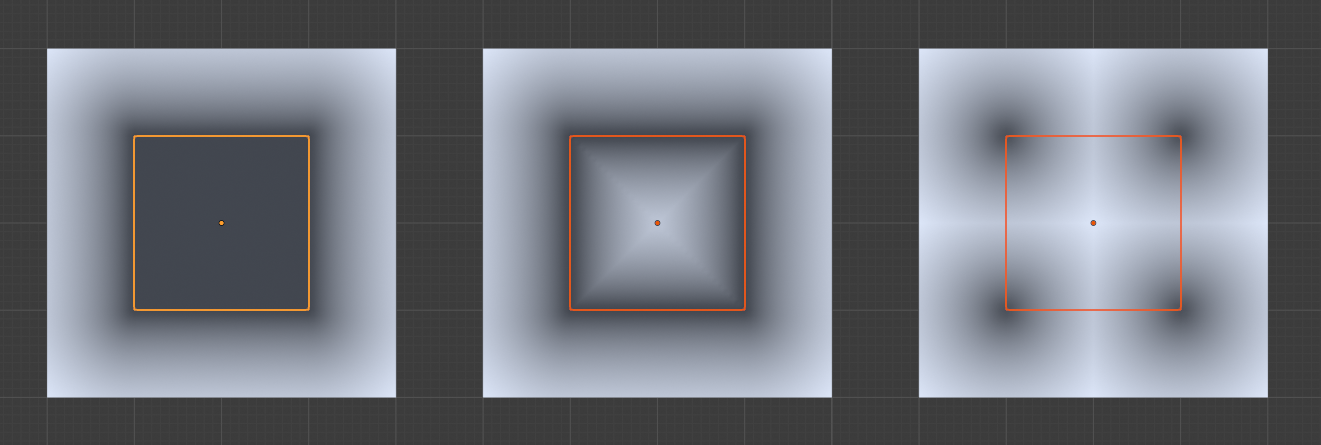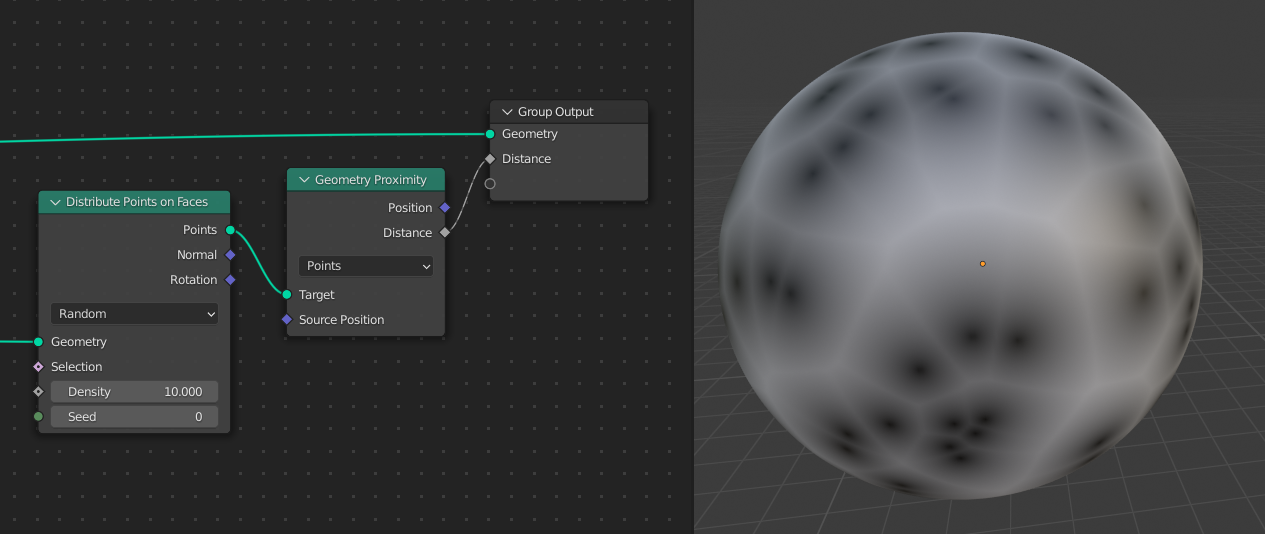# Geometry Proximity Node

The Geometry Proximity node computes the closest location on the target geometry.

Tips

The Map Range Node is often helpful to use with the distance output of this node to create a falloff with a maximum distance.

## Inputs

Target

Standard geometry input.

Source Position

The given position to calculate the closest location on the target.

## Properties

Target Element
Faces

Calculate the closest point anywhere on the faces of the target’s mesh geometry.

Edges

Calculate the closest point anywhere on the edges of the target’s mesh geometry.

Points

Calculate the closest point or vertex on the target geometry. This mode is usually the fastest. This mode works for both point cloud and mesh geometry, the other modes only work for meshes.

## Outputs

Position

Closest location on the surface of the target mesh, or the closest point in the target point cloud in Points mode.

Distance

Distance (as floating-point value) from the source position to the closest location in the target.

## Examples

The different modes of the node: faces, edges and points. In this example the Geometry Nodes modifier is added on the target plane. Note that the larger plane is subdivided and the smaller plane is not.The three target element modes: faces, edges, and points.Points distributed on a sphere used as a target for a distance used in a shader.